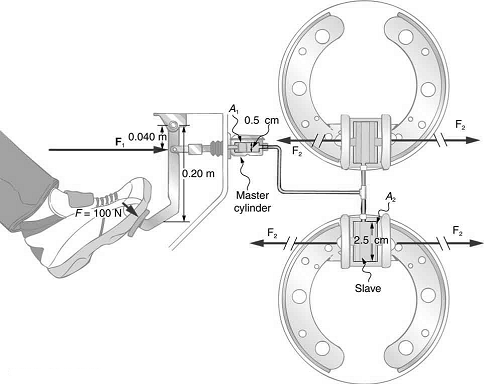Chapter 11, Problem 24PE

Chapter
Section
Textbook Problem

# How much pressure is transmitted in the hydraulic system considered in Example 11.6? Express your answer in pascals and in atmospheres.

To determine

How much pressure is transmitted in the hydraulic system considered in the figure?Explanation

Given info:

F=100Nd1=0.5cm=5×103m

Formula used:

Pressure,

P=FA , F is the normal force and A is the area of force acting.

Calculation:

The torque in the hinge is zero,

F×0.2+F1×0.04=0F1=500NP=F1

### Still sussing out bartleby?

Check out a sample textbook solution.

See a sample solution

#### The Solution to Your Study Problems

Bartleby provides explanations to thousands of textbook problems written by our experts, many with advanced degrees!

Get Started

## Additional Science Solutions

#### Find more solutions based on key concepts